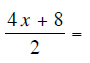## FORM 1 ALGEBRA – MATHEMATICS TEST

FORM 1 ALGEBRA – MATHEMATICS TEST

1. For each of these questions, choose the option (A, B, C or D) that is CORRECT.

5(x + y) − 3(x y) =

2. Choose the option (A, B, C or D) that is CORRECT.Question 1 of 10